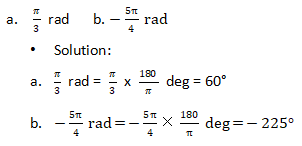# 国际课程提前学，A-Level数学之三角函数1：角的测量

Angles角

An angle is determined by a rotation of a ray about its endpoint. The endpoint of the ray is the vertex of the angle. The stating position of the ray is the initial side of the angle, and the position after rotation is the terminal side.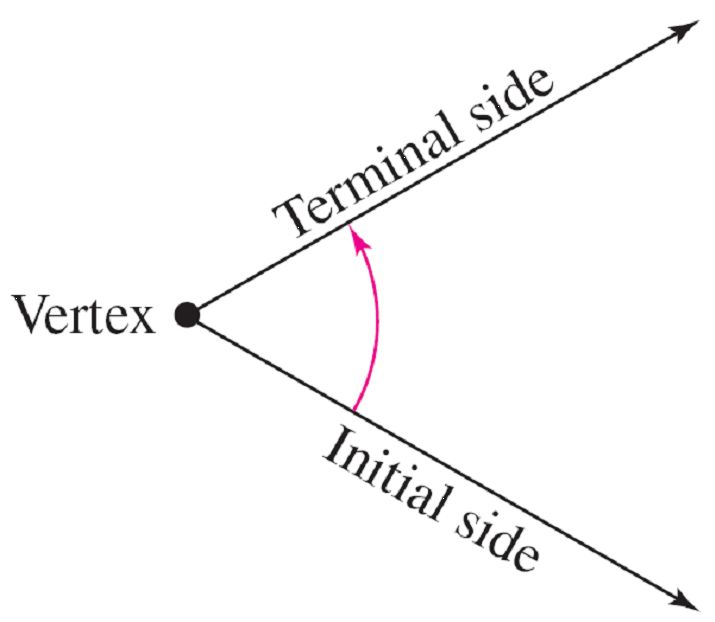angle is in standard position 角的标准位置

This perception of an angle fits a coordinate system in which the origin
is the vertex and the initial side coincides with the positive x-axis.

Such an angle is in standard position.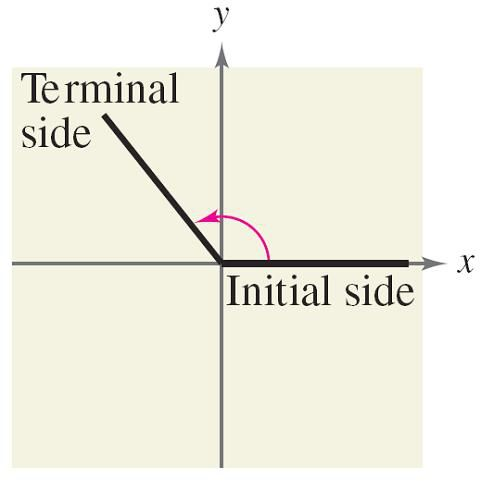Positive and negative angles角的正负

Positive angles are generated by counterclockwise rotation, and
negative angles by clockwise rotation.

Coterminal 终边相同的角

note that angles a and b have the same initial and
terminal sides. Such angles are coterminal.

a和b角有相同的初始边和终边，这样特点的角是终边相同的角。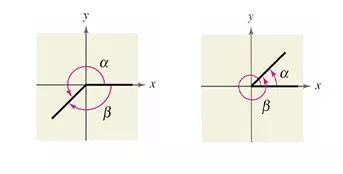Degree angles角的测量（度数）

A way to measure angles is in terms of degrees, denoted by the symbol °.

A measure of one degree (1°) is equivalent to a rotation of a complete revolution about the vertex.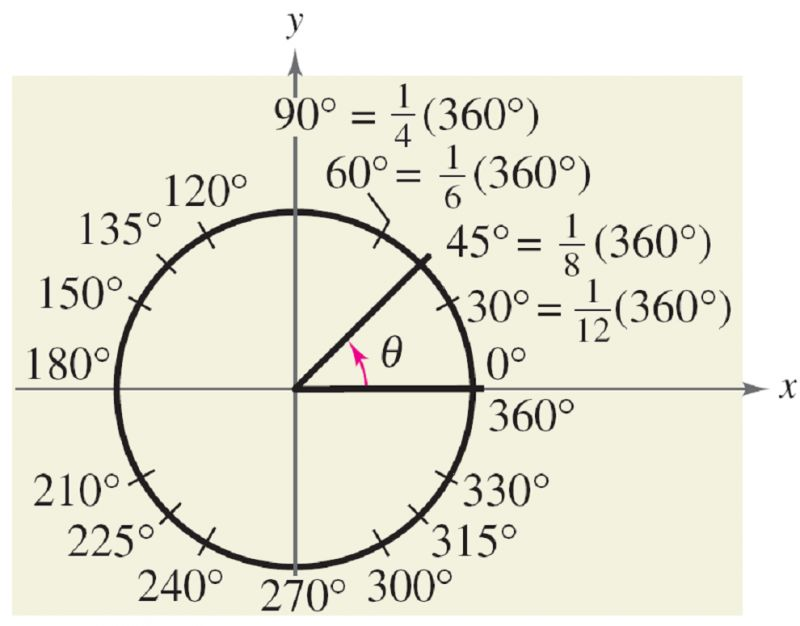he second way of measure of an angle is determined by the amount of rotation from the initial side to the terminal side，this way to measure angles is in radians. This type of measure is especially useful in calculus.

To define a radian, you can use a central angle of a circle, one whose vertex is the center of the circle.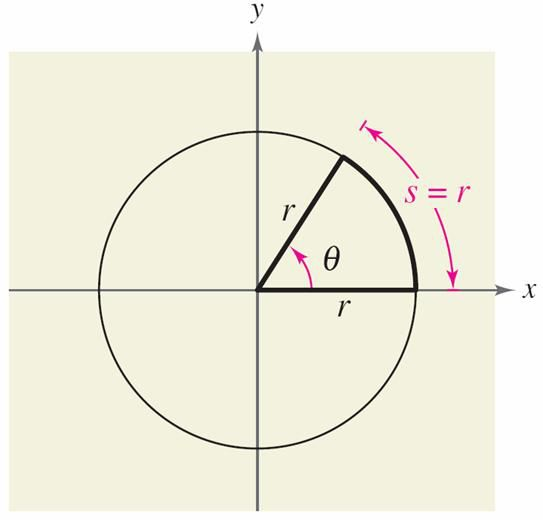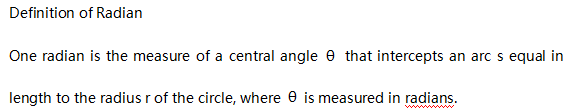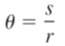These and other common angles are shown in the figure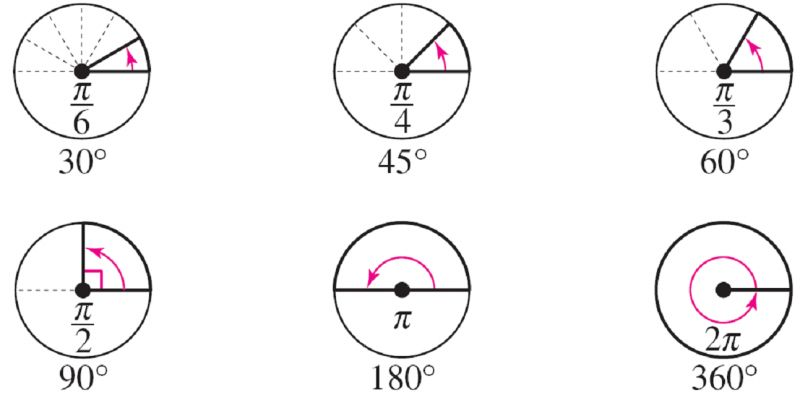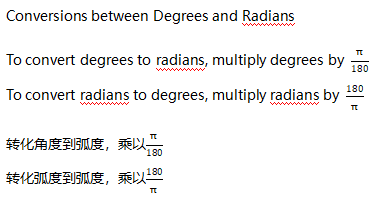Example 1 Convert from degrees to radians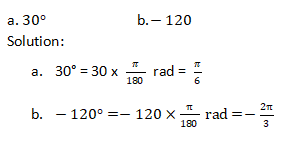Example 2 Convert from radians to degrees